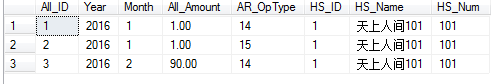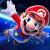# 关于sql 的统计，一个字段，不同类型

Mr_K 发布于 2016/06/06 12:180

```SELECT HS_Name
, SUM(All_Amount) as out_amount
FROM TableName
WHERE AR_OpType == 14
GROUP BY HS_Name```

```SELECT HS_Name
, SUM(All_Amount) as out_amount
FROM TableName
WHERE AR_OpType == 15
GROUP BY HS_Name```

```SELECT HS_Name
, in_amount
, out_amount
, NVL(in_amount) - NVL(out_amount) as result;
FORM (
SELECT HS_Name
, SUM(All_Amount) as in_amount
FROM TableName
WHERE AR_OpType == 14
GROUP BY HS_Name
) A FULL JOIN (
SELECT HS_Name
, SUM(All_Amount) as out_amount
FROM TableName
WHERE AR_OpType == 15
GROUP BY HS_Name
) B
ON ( A.HS_Name == B.HS_Name )```

result列就是结果，请将HS_Name替换成你需要的三个列，NVL函数替换为SQL Server中对应的函数体。
0
A

```select Year,Month,HS_ID,HS_Name,HS_Num,
sum(all_amount*(29-ar_optype*2)) amt
from tableName
where condition
--如果ar_optype 包含其他类型, 那么这里加(ar_optype=14 or ar_optype=15)
group by Year,Month,HS_ID,HS_Name,HS_Num```

0```SELECT YEAR,MONTH,HS_ID,HS_NAME,HS_NUM,
SUM(CASE WHEN AR_OPTYPE = 14 THEN ISNULL(ALL_AMOUNT,0)
WHEN AR_OPTYPE = 15 THEN ISNULL(ALL_AMOUNT,0)*-1
ELSE 0
END) TOTAL_AMOUNT
FROM TAB
WHERE AR_OPTYPE IN (14,15)
GROUP BY YEAR,MONTH,HS_ID,HS_NAME,HS_NUM;```